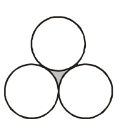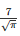Courses

# Test: Area Related To Circles- 4

## 25 Questions MCQ Test Mathematics (Maths) Class 10 | Test: Area Related To Circles- 4

Description
This mock test of Test: Area Related To Circles- 4 for Class 10 helps you for every Class 10 entrance exam. This contains 25 Multiple Choice Questions for Class 10 Test: Area Related To Circles- 4 (mcq) to study with solutions a complete question bank. The solved questions answers in this Test: Area Related To Circles- 4 quiz give you a good mix of easy questions and tough questions. Class 10 students definitely take this Test: Area Related To Circles- 4 exercise for a better result in the exam. You can find other Test: Area Related To Circles- 4 extra questions, long questions & short questions for Class 10 on EduRev as well by searching above.
QUESTION: 1

Solution:
QUESTION: 2

Solution:
QUESTION: 3

### Three circles of equal radii touch each other as shown in figure. The radius of each circle is 1 cm. What is the area of shaded region?Solution:
QUESTION: 4

The area of a circle is 38.5 sq. cm. Its circumference is

Solution:

Area of circle = 38.5 sq cm
That is πr2 = 38.5
r2 = 38.5 × (7/22) = 12.25
Therefore, r = 3.5 cm
Circumference of circle = 2πr
= 2 x (22/7) x 3.5
= 22 cm

QUESTION: 5

The circumference of a circle whose diameter is 4.2cm is

Solution:
QUESTION: 6

The length of the wire is 66m. The number of circles of circumference 13.2cm can be made from the wire is

Solution:
QUESTION: 7

The number of rounds that a wheel of diameter 7/11 m will make in going 4 km is

Solution:
QUESTION: 8

The circumference of a circle exceeds its diameter by 120cm, then its radius is

Solution:
QUESTION: 9

The area of a square inscribed in a circle of diameter ‘d’ is

Solution:
QUESTION: 10

The distance covered by a circular wheel of diameter ‘d’ in 100 revolutions is

Solution:
QUESTION: 11

The radius of the circle whose area is equal to the sum of the areas of the two circles of radii 24cm and 7cm is

Solution:
QUESTION: 12

The radius of the circle whose circumference is equal to the sum of the circumferences of the two circles of radii 24cm and 7cm is

Solution:
QUESTION: 13

The perimeter of a semicircular protractor whose radius is 7cm is

Solution:
QUESTION: 14

If the radius of the circle iscm, then its area is

Solution:
QUESTION: 15

The area of a circle is 2464 sq. cm, then its diameter is given by

Solution:
QUESTION: 16

If ‘r’ is the radius of a circle, then its perimeter is given by

Solution:
QUESTION: 17

The area of the square that can be inscribed in a circle of radius 12cm is

Solution:
QUESTION: 18

The area of the circle that can be inscribed in a square of side 10cm is

Solution:
QUESTION: 19

If the perimeter of a circle is equal to that of a square, then the ratio of their areas is

Solution:
QUESTION: 20

The radius of a circle whose circumference is equal to the sum of the circumferences of the two circles of diameters 36cm and 20cm is

Solution:
QUESTION: 21

If the sum of the circumferences of two circles with radii R1and R2 is equal to the circumference of a circle of radius R, then

Solution:
QUESTION: 22

If the circumference of a circle and the perimeter of a square are equal, then

Solution:
QUESTION: 23

The diameter of a circular park is 17.5 m. It is surrounded by a path of width 3.5 m. The area of the path is given by

Solution:
QUESTION: 24

The radii of two concentric circles are 19cm and 16cm. The area of the ring enclosed between them is

Solution:
QUESTION: 25

If the difference between the circumference and radius of a circle is 37 m, then the circumference of the circle is given by

Solution: